# DAV Class 6 Maths Chapter 2 Brain Teasers Solutions

The DAV Class 6 Maths Book Solutions Pdf and DAV Class 6 Maths Chapter 2 Brain Teasers Solutions of Factors and Multiples offer comprehensive answers to textbook questions.

## DAV Class 6 Maths Ch 2 Brain Teasers Solutions

Question 1A.

(a) Six bells commence tolling together and toll at intervals of 2, 4, 6, 8, 10 and 12 seconds respectively. After how many minutes will they toll together again?
(i) 5 minutes
(ii) 6 minutes
(iii) 4 minutes
(iv) 2 minutes
Solution:
(iv) 2 minutesLCM = 2 × 2 × 3 × 2 × 5
= 120 sec
= 2 min
So, six bells will toll together after 2 min.
Hence, (iv) is the correct option.

(b) The HCF of two numbers is 11 and their LCM is 7700. If one of the numbers is 275, then the other number is
(i) 279
(ii) 283
(iii) 308
(iv) 318
Solution:
(iii) 308

It is given that HCF = 11,
LCM = 7700,
First number = 275
We know that
HCF × LCM = Product of numbers
or, HCF × LCM = First number × second number
11 × 7700 = 275 × second number
or $$\frac{11 \times 7700}{275}$$ = second number
∴ 308 = second number
Hence, (iii) is the correct option.(c) The greatest possible length which can be used to measure exactly the lengths 7m, 3m 85 cm, 12 m 95 cm is—
(i) 35 cm
(ii) 25 cm
(iii) 15 cm
(iv) 42 cm
Solution:
(i) 35 cm
To find the maximum length, we have to find HCF
First length = 7 m = 700 cm
Second length = 3m 85 cm = 385 cm
Third length = 12 m 95 cm = 1295 cm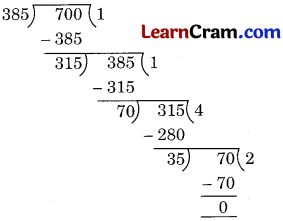So, HCF of 385 and 700 is 35.
Now, let us find the HCF of 35 and the third number, i.e., 1295 cm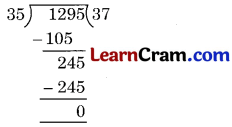HCF = 35 cm
Hence, (i) is the correct option.

(d) 252 can be expressed as a product of prime as—
(i) 2 × 2 × 3 × 3 × 7
(ii) 2 × 2 × 2 × 3 × 7
(iii) 3 × 3 × 3 × 3 × 7
(iv) 2 × 3 × 3 × 3 × 7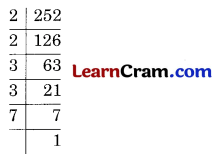∴ 252 = 2 × 2 × 3 × 3 × 7
Hence, (i) is the correct option.

(e) Which of the following is a factor of every natural number?
(i) 1
(ii) 0
(iii) – 1
(iv) any number
Solution:
2 × 1 = 2, 3 × 1 = 3, 4 × 1 = 4, .. so on.
∴ 1 is a factor of every natural number.
Hence, (i) is the correct option.(a) Find the highest common factor of 36 and 84.
Solution:
We can find the HCF of 36 and 84 by continued division method.
84 is greater than 36, so 84 will be the dividend∴ HCF = 12

(b) How many factors does 36 have?
Solution:
1 × 36 = 36
2 × 18 = 36
3 × 12 = 36
4 × 9 = 36
6 × 6 = 36
Factors of 36 are 1, 2, 3, 4, 6, 9, 12, 36
which are nine (9) in number.
So, 36 have 9 factors.(c) Express 132 as the sum of two odd primes.
Solution:
71 + 61

(d) What should be added to 4057 to make it divisible by 9?
Solution:In order to make 7 divisible by 9, we have to add 2 in 7.
So, we have to add 2 to 4057 to make it divisible by 9.

(e) Find the HCF of 95, 105 and 115 by continued division.
Solution:
HCF by continued division method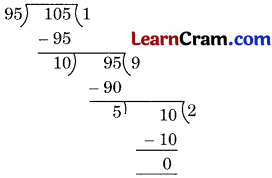So, HCF of 95 and 105 is 5.
Now, let us find the HCF of 5 and the third number, i.e., 115
∴ HCF = 5.Question 2.
Are 32 and 34 co-prime numbers? Why?
Solution:
No, because 2 is the common factor of 32 and 34.

Question 3.
Write any four twin primes between 50 and 110.
Solution:
(59 and 61), (71 and 73), (101, 103), (107, 109)

Question 4.
Express the greatest 3-digit number as a product of primes.
Solution:
The greatest 3-digit number is 999∴ 999 = 33 × 37Hence, required product = 33Question 5.
Express the smallest 5-digit number as a product of primes.
Solution:
5-digit smallest number = 10000∴ 10000 = 24 × 54
Hence, the required product = 24 × 54.

Question 6.
State which of the following numbers are divisible by both 3 and 9?
(a) 235674
(b) 78015
Solution:
(a) 235674 Sum of the digits = 2 + 3 + 5 + 6 + 7 + 4 = 27
27 is divisible by 3 and 9 both.
Hence, 235674 is divisible by 3 and 9.

(b) 78015
Sum of the digits = 7 + 8 + 0 + 1 + 5 = 21
21 is divisible by 3 but not by 9.
Hence, 21 is not divisible by 3 and 9 both.

Question 7.
Test which of the following numbers are divisible by 11.
(a) 147246
(b) 2352825
Solution:
(a) 147246
Sum of the digits in odd places
1 + 7 + 4 = 12
Sum of the digits in even places 4 + 2 + 6 = 12
Difference = 12 – 12 = 0
Hence, 147246 is divisible by 11.

(b) 2352825
Sum of digits in odd places
2 + 5 + 8 + 5 = 20
Sum of digits in even places
3 + 2 + 2 = 7
Difference 20 – 7 = 13
Hence, 2352825 is not divisible by 11.Question 8.
What least number should be subtracted from the following numbers to make them divisible by 3?
(a) 2825
(b) 856291
Solution:
(a) Sum of digits of 2825 = 2 + 8 + 2 + 5 = 17
17 – 2 = 15 which is divisible by 3
Hence, the required number = 2

(b) Sum of digits of 856291
= 8 + 5 + 6 + 2 + 9+1 = 31
31 – 1 = 30
which is divisible by 3
Hence, the required number = 1

Question 9.
What number should be added to the following to make them divisible by 9?
(a) 42724
(b) 39065
Solution:
(a) Sum of the digits of 42724 = 4 + 2 + 7 + 2 + 4 = 19
19 + 8 = 27 which is divisible by 9
Hence, the required number = 8

(b) The sum of the digits of 39065 = 3 + 9 + 0 + 6 + 5 = 23
23 + 4 = 27 which is divisible by 9
Hence, the required number = 4

Question 10.
Replace the blank in 625___ with the least number, so that the number is divisible by 11.
Solution:
625___
Sum of digits of odd places = 6 + 5 = 11
Sum of digits of even places = 2 + ___ = 11
∴ ___ = 11 – 2 = 9
Hence, the required least number = 9.Question 11.
Write any two numbers which are
(a) divisible by 3 but not 9
(b) divisible by 5 but not 10
(c) divisible by both 4 and 8
(d) divisible by 2, 4, 8
Solution:
(a) 2334
Sum of the digits = 2 + 3 + 3 + 4 = 12 which is divisible by 3 but not 9. Now take 65382
Sum of the digits = 6 + 5 + 3 + 8 + 2 = 24 which is divisible by 3 but not 9.
Hence, 2334 and 65382 are the numbers which are divisible by 3 but not 9.
(b) 255 and 625 are the numbers which are divisible by 5 but not 10.
(c) 2424 and 8048 are the two numbers which are divisible by 4 and 8.
(d) Common multiples of 2, 4 and 8 are 8 and 16.
Hence 8 and 16 are the numbers which are divisible by 2, 4 and 8.

Question 12.
Find the HCF of 1624, 522, 1276.
Solution:
Here, 1624 > 1276 > 522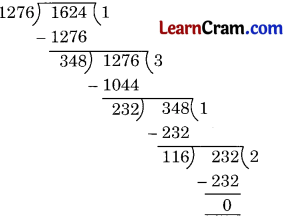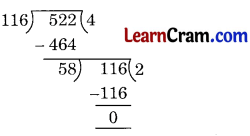HCF of 1276 and 1624 = 116
Hence, required HCF = 58Question 13.
Find the LCM of 198, 135, 108, 54.
Solution: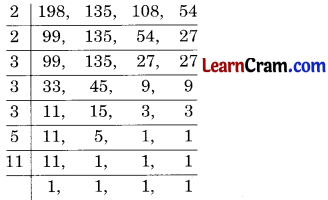LCM = 2 × 2 × 3 × 3 × 3 × 5 × 11
= 5940
Hence, LCM = 5940.

Question 14.
The HCF and LCM of two numbers are 13 and 1989 respectively. If one number is 117, find the other.
Solution:
HCF × LCM = Product of two numbers
13 × 1989 = 117 × other number
∴ Other number =Hence, the other number = 221.

Question 15.
Can two numbers have 15 as HCF and 350 as LCM? Why?
Solution:
No, because 350 is not completely divisible by 15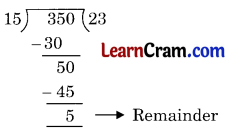### DAV Class 6 Maths Chapter 2 HOTS

Question 1.
Find the greatest number of four digits which is divisible by 15, 20 and 25.
Solution:
LCM of 15, 20 and 25 is given by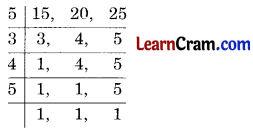∴ LCM = 5 × 3 × 4 × 5 = 300
Greatest four digit number = 9999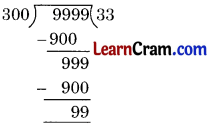∴ Greatest number of four digits, which is divisible by 15, 20 and 25 = 9999 – 99 = 9900.Fill in the blanks:

(a) If the difference of the sum of the digits at odd places and the sum of the digits at even places is divisible by 0 or 11, then the number is divisible by _________.
Solution:
11

(b) If a and b are any two co-prime numbers, then their HCF is _________.
Solution:
1

(c) Product of two numbers = HCF × _________
Solution:
LCM

(d) LCM of the numbers must be _________ by their HCF.
Solution:
divisible

(e) 48351 is divisible by 3 but not by _________.
Solution:
9

(f) If a number is divisible by 6 then it must be divisible by _________ and _________.
Solution:
2, 3

(g) 1331 is divisible by _________.
Solution:
11

(h) 15 and 35 are divisible by _________.
Solution:
5

(i) LCM of 6 and 7 = 6 × ___.
Solution:
7Question 2.
Which of the following numbers are divisible by 11?
(a) 2 4 8 6 3 3
(b) 6 5 8 9 1 1
(c) 8 2 5 6 8 3
(d) 9 9 9 9 9
(a) Sum of the digits at odd places = 2 + 8 + 3 = 13
Sum of the digits at even places = 4 + 6 + 3 = 13
Difference = 13 – 13 = 0
Hence, it is divisible by 11.

(b) Sum of the digits at odd places = 6 + 8 + 1 = 15
Sum of the digits at even places = 5 + 9 + 1 = 15
Difference = 15 – 15 = 0
Hence, it is divisible by 0.

(c) Sum of digits at odd places = 8 + 5 + 8 = 21
Sum of digits at even places = 2 + 6 + 3= 11
Difference = 21 – 11= 10
Hence, it is not divisible by 11.

(d) The sum of the digits at odd places = 9 + 9 + 9 = 27
Sum of the digits at even places = 9 + 9 = 18
Difference = 27 – 18 = 9
Hence, it is not divisible by 11.Question 3.
Find the greatest number that can exactly divide 140, 170 and 155 leaving remainder 5 in each case.
Solution:
H.C.F. of 140, 170 and 155
Here, we have to find the HCF of 140 – 5 = 135, 170 – 5 = 165 and 155 – 5 = 150Hence, HCF = 15 and
the required greatest number = 15.

Question 4.
Find the Prime factorisation of the following;
(a) 132
Solution: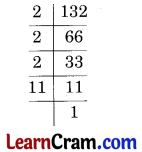Prime factors of 132 = 2 × 2 × 2 × 3 × 11

(b) 3600
Solution:Prime factors of 3600 = 2 × 2 × 2 × 2 × 3 × 3 × 5 × 5

(c) 1176
Solution: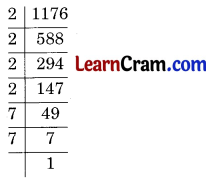Prime factor’s of 1176 = 2 × 2 × 2 × 3 × 7 × 7Question 4.
Find the LCM of 80, 120 and 135
Solution: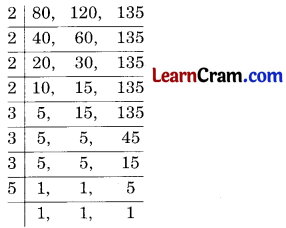LCM = 2 × 2 × 2 × 2 × 3 × 3 × 3 × 5 = 4560
Hence, the required LCM = 4560.

Question 5.
Find the smallest number which when divided by 25,45 and 60 leaves remainder 5 in each case.
Solution:
LCM of 25, 45 and 60LCM = 2 × 2 × 3 × 3 × 5 × 5 = 900
Hence, the required number = 900 + 5 = 905.

Question 6.
Three DTC Buses stop after 50, 120 and 135 km respectively. If the above buses leave together, after how many kilometers will they stop together if they start at 2 p.m?
Solution:
LCM of 50, 120 and 135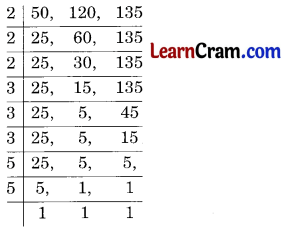LCM = 2 × 2 × 2 × 3 × 3 × 3 × 5 × 5 = 540 kilometers
Hence, the required distance = 540 km.Question 7.
Find whether the following numbers are prime or composite (show by finding the factors):
(a) 9
(b) 48
(c) 89
(d) 96
(e) 78
(f) 101
Solution:
(a) 9 = 1 × 9, 3 × 3
The factors of 9 are 1, 3, 9
Hence, it is a composite number.

(b) 48 = 1 × 48, 2 × 24, 3 × 16, 4 × 12, 6 × 8
The factors of 48 are 1, 2, 3, 4, 6, 8, 12, 16, 24 and 48
Hence, it is composite number.

(c) 89 = 1 × 89
89 has only two factors 1 and itself.
Hence, it is a prime number.

(d) 96 = 1 × 96, 2 × 48, 3 × 32, 4 × 24, 6 × 16, 8 × 12
The factors of 96 are 1, 2, 3, 4, 6, 8, 12, 16, 24, 32, 48, 96
Hence, it is a composite number.

(e) 78 = 1 × 78, 2 × 39, 3 × 26, 6 × 13
The factors of 78 are 1, 2, 3, 6, 13, 26, 39, 78
Hence, it is a composite number.

(f) 101 = 1 × 101
It has only two factors 1 and itself.
Hence, it is prime number.